# Isosceles Trapezoid

Tim and Jack were identifying the different types of quadrilaterals. They could identify the squares, rectangles, kites, and rhombuses. Except for one figure, which they couldn't fit in the quadrilateral chart, they identified all others.

The quadrilateral they had failed to identify had 1 pair of equal sides and another pair of parallel sides. Tim said that the figure should be placed under parallelograms as it had one pair of parallel sides, but Jack refused.

Jack was convinced that it was a type of trapezoid.

Jack was right, but what he did not know was that this trapezoid is called the isosceles trapezoid. In this lesson, you will know more about both, the isosceles and the non-isosceles trapezoid. You will also learn the isosceles trapezoid area formula, as well as calculating the isosceles trapezoid’s perimeter.

Check out the interactive simulations to know more about the lesson and try your hand at solving a few interesting practice questions at the end of the page.

## Lesson Plan

 1 What is Isosceles Trapezoid? 2 Important Notes on Isosceles Trapezoid 3 Solved Examples on Isosceles Trapezoid 4 Challenging Questions on Isosceles Trapezoid 5 Interactive Questions on Isosceles Trapezoid

## What is Isosceles Trapezoid?

A trapezoid is a quadrilateral in which one pair of opposite sides are parallel.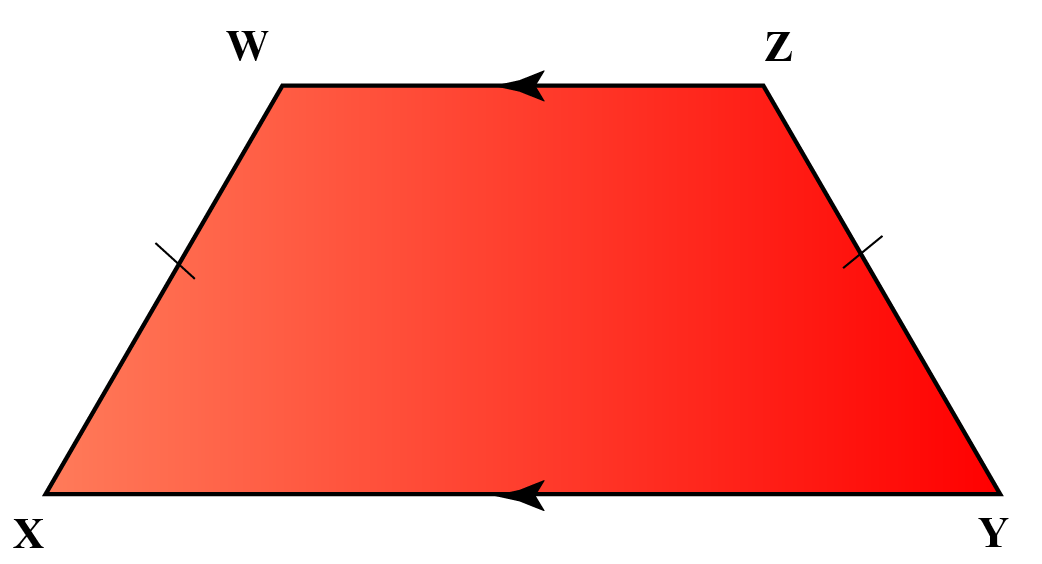Now, as you can see in this diagram $$XY$$ and $$WZ$$ are parallel to each other and are called bases.

Meanwhile, the sides that are not parallel to each other are called legs.

In the above image, $$WX$$ and $$YZ$$ are the legs of the trapezoid.

Now if the legs of the trapezoid are equal in length, then it is called an isosceles trapezoid.

## What Are the Properties of Isosceles Trapezoid?

Except for the common properties of all trapezoids, the isosceles trapezoids have some special properties.

### Property 1

It has an axis of symmetry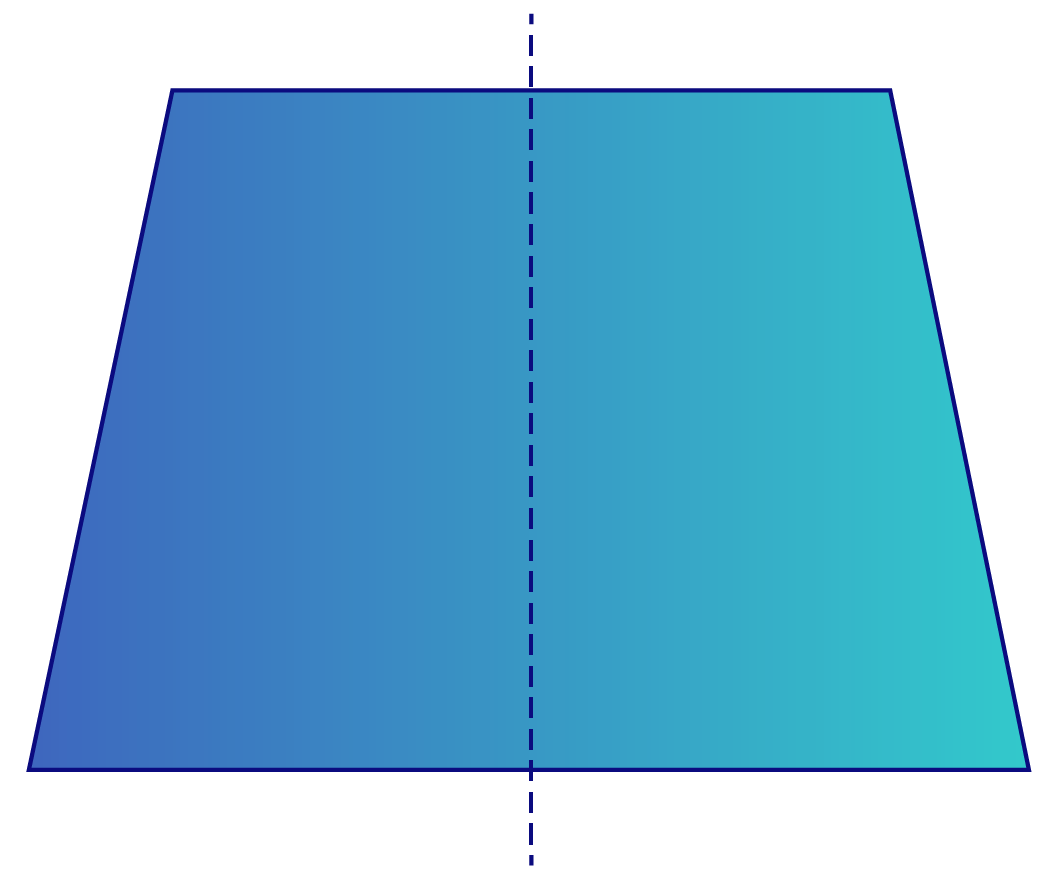### Property 2

Both the diagonals are of equal length.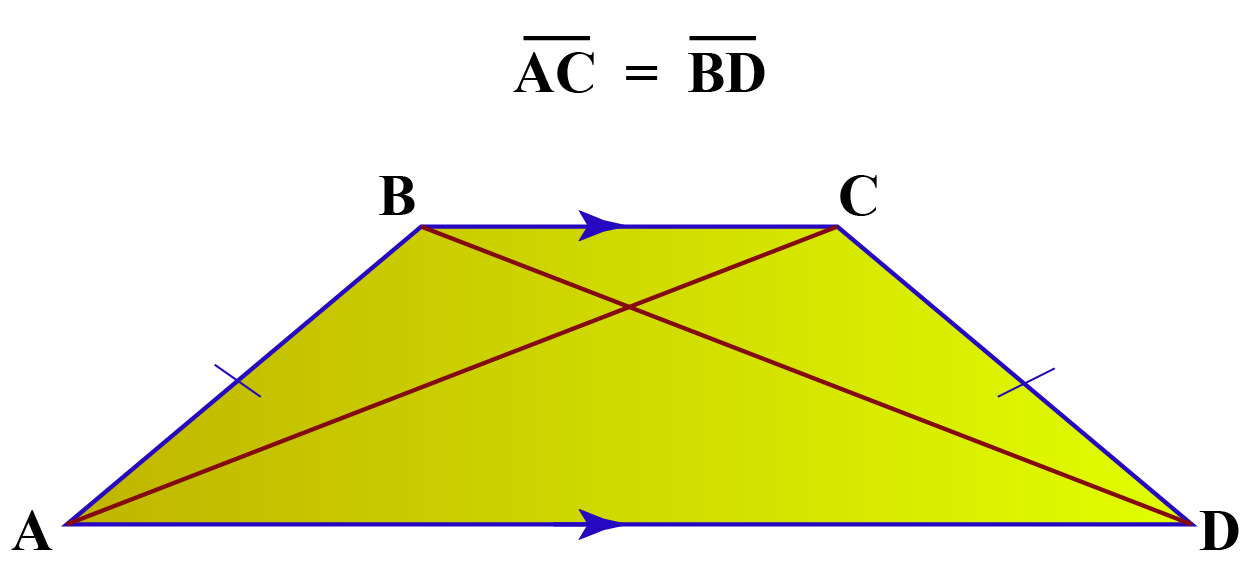### Property 3

The area of an isosceles trapezoid is $A = \dfrac{{a+b }}{2}\times h$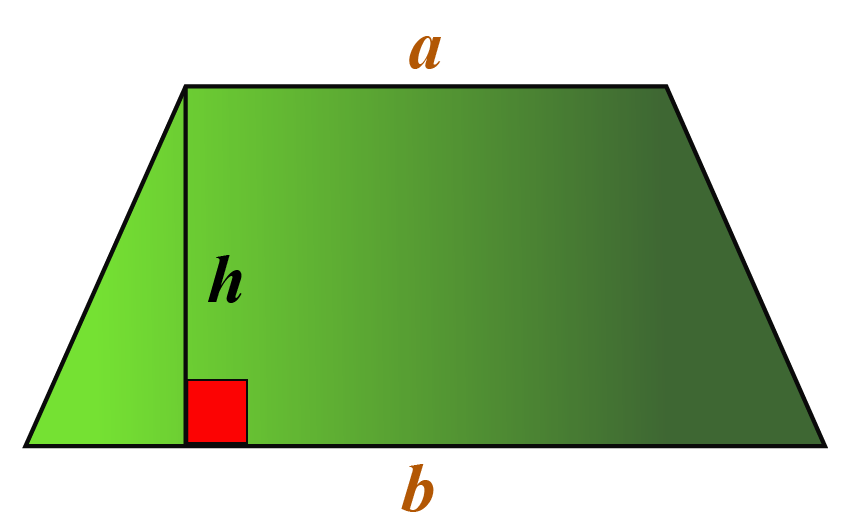### Property 4

The base angles are equal in measure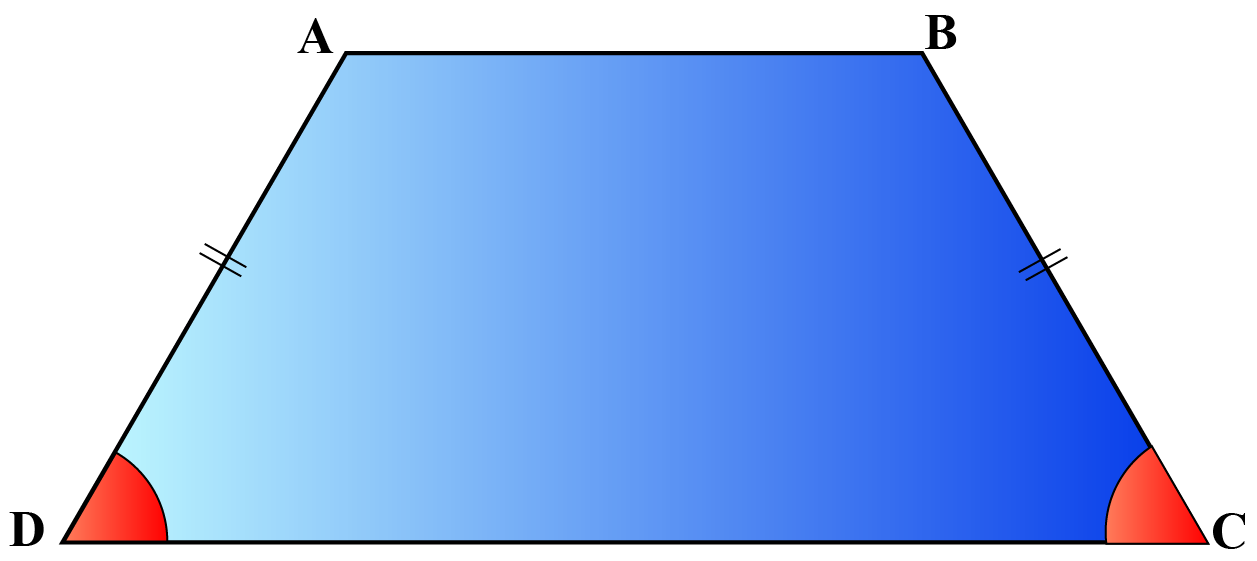$$\angle ADC = \angle BCD$$

More Important Topics
Numbers
Algebra
Geometry
Measurement
Money
Data
Trigonometry
Calculus